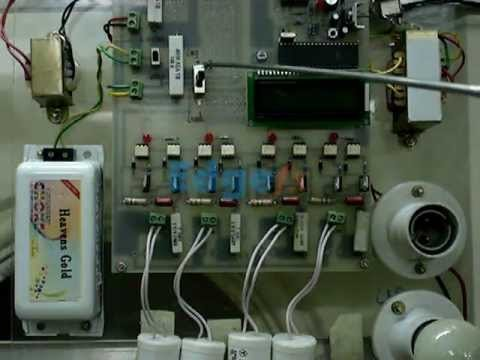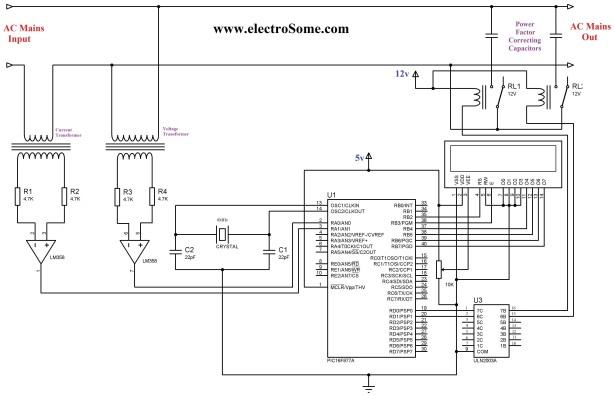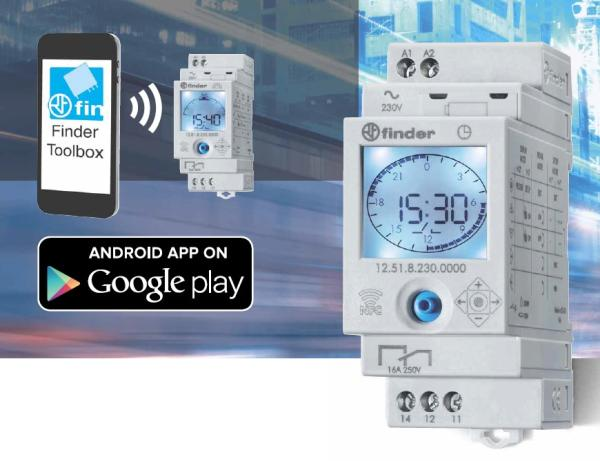# automatic power factor controller using microcontroller

The 230 V, 50 Hz is step downed using voltage transformer and current transformer is used to extract the waveforms of current. The output of the voltage transformer is proportional to the voltage across the load and output of current transformer is proportional to the currentG‚throughG‚the load. These waveforms are fed to Voltage Comparators const visit page.

ructedG‚usingG‚LM358G‚op-amp. Since it is a zero crossing detector, its outputG‚changesG‚during zero crossing of the current and voltage waveforms. These outputs are fed to the PIC which does the further power factor calculations. PIC 16F877A microcontroller is the heart of this Automatic Power Factor Controller, it find, displays andG‚controlsG‚the Power Factor.To correct power factor, first we need to find the current power factor. It can be find by taking tangent of ratio of time between zero crossing of current and voltageG‚waveformsG‚and two successive zero crossing of voltage waveform. Then it displays the calculated power factor in the 16G—2 LCD Display andG‚switchesG‚ON the capacitors if required. When load is connected the power factor is calculated by the PIC microcontroller. If the calculated power factor is less than 0. 9 then the relay switches on the capacitor. The relays are switched using ULN2003 which is basically a driver IC. ULN2003 consists of seven DARLINGTON PAIRS. The current lead in capacitor compensates the corresponding current lag which is usually present in loads. Hence the phase difference between the current and voltage will be reduced. Power Factor Correcting capacitor connected parallel to load through relay, if the relay is energized by microcontroller it will connect G‚the capacitor parallel with load, if relay deenergized it will remove the capacitor from the load. When the resistive load is on the power factor will be near to unity so the microcontrollerG‚doesn`tG‚energize the relay coil. When the inductive load is on the power factor decrease now the microcontroller energize the relay coil in order to compensate the excessive reactive power. Hence according to the load the power factor is corrected. //LCD Module Connections sbit LCD_RS at RB4_bit; sbit LCD_EN at RB5_bit; sbit LCD_D4 at RB0_bit; sbit LCD_D5 at RB1_bit; sbit LCD_D6 at RB2_bit; sbit LCD_D7 at RB3_bit; sbit LCD_RS_Direction at TRISB4_bit; sbit LCD_EN_Direction at TRISB5_bit; sbit LCD_D4_Direction at TRISB0_bit; sbit LCD_D5_Direction at TRISB1_bit; sbit LCD_D6_Direction at TRISB2_bit; sbit LCD_D7_Direction at TRISB3_bit; //End LCD Module Connections int powerFactor() { int a=0, b=0, t=0, x=0; float tm, pf; TMR1L=0; TMR1H=0; do { if(PORTA. F0 = 1) T1CON. F0 = 1; else if(PORTA. F0 = 0 && T1CON. F0 = 1) { T1CON. F0 = 0; break; } }while(1); a = (TMR1L | (TMR1H<<8) * 2; TMR1L=0; TMR1H=0; do { if(PORTA. F0 = 1) { T1CON. F0=1; if(PORTA. F1=1) { T1CON. F0=0; break; } } }while(1); b = TMR1L | (TMR1H<<8); tm = (float)b/a; pf = cos(tm*2*3. 14); x=abs(ceil(pf*100); return x; } void main() { char c[]=”0. 00″; int a, b, d, x, f, e; float tm, pf; Lcd_Init();Lcd_Cmd(_LCD_CURSOR_OFF); // Cursor off ADCON1 = 0x08; // To configure PORTA pins as digital TRISA. F0 = 1; // Makes First pin of PORTA as input TRISA. F1 = 1; //Makes Second pin of PORTA as input TRISD. F0 = 0; //Makes Fist pin of PORTD as output TRISD. F1 = 0; //Makes Second pin of PORTD as output while(1) { a = powerFactor(); Delay_us(50); b = powerFactor(); Delay_us(50); d = powerFactor(); Delay_us(50); e = powerFactor(); Delay_us(50); f = powerFactor(); x = (a+b+d+f+e)/5; c=x%10 + 0x30; x=x/10; c=x%10 + 0x30; x=x/10; c=x%10 + 0x30; Lcd_Out(1, 1, “Power Factor”); Lcd_Out(2, 1, c); if(x<90) { PORTD. F0 = 1; PORTD. F0 = 1; Delay_ms(2000); } else { PORTD. F0 = 0; PORTD. F0 = 0; } Delay_ms(250); } } The function powerFactor() will find the current power factor by using the Timer 1 module of PIC 16F877a. Power Factor may be fluctuating, so to avoid it we will find power facto

READ  PIC microcontroller power supply circuit by Chayapol

For more detail: automatic power factor controller using microcontroller

#### Current Project / Post can also be found using:

• automatic power factor correction using proteus
• simple power factor measurement pic microcontroller with mikroc code
• Power factor correction circuit Using pic micro controller
• power factor improvement using pic microcontroller with source code